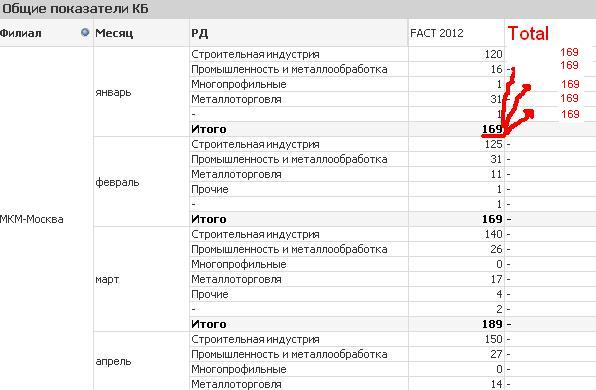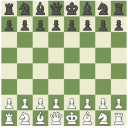# QlikView App Dev

Discussion Board for collaboration related to QlikView App Development.

Announcements
Customer & Partners, DEC. 9, 11 AM ET: Qlik Product & Strategy Roadmap Session: Data Analytics REGISTER NOW
cancel
Showing results for
Did you mean:Not applicable

## total field

Hi !

I have table with expression (FACT 2012):

sum(if((Aggr(sum({<year={"2012"}, bg_t={'*'}, bg_t-={'b\g}, Holding=>} Quantity),AffiliateID, Holding,mes))>0 ,1,0))

How can i get field with total sum?1 Solution

Accepted SolutionsMVP

Try adding total <Dim1,Dim2> to the sum expression. Replace Dim1 and Dim2 with the first two dimensions of your pivot table.

sum(total <Dim1,Dim2>  if((Aggr(sum({<year={"2012"}, bg_t={'*'}, bg_t-={'b\g}, Holding=>} Quantity),AffiliateID, Holding,mes))>0 ,1,0))

See attached example for how it works.

talk is cheap, supply exceeds demand
7 RepliesNot applicable
AuthorMVP

Try adding total <Dim1,Dim2> to the sum expression. Replace Dim1 and Dim2 with the first two dimensions of your pivot table.

sum(total <Dim1,Dim2>  if((Aggr(sum({<year={"2012"}, bg_t={'*'}, bg_t-={'b\g}, Holding=>} Quantity),AffiliateID, Holding,mes))>0 ,1,0))

See attached example for how it works.

talk is cheap, supply exceeds demandNot applicable
Author

Thank you, Gysbert for your help!

I put exemple in you attachment, please lookMVP

Hi,

Try this expression

sum(Total<Year, Product, Region>if((Aggr(sum({<year={"2008"}, Product={'*'}, product-={'Banana'}, Holding=>} Sales),Year, Product,Month))>0 ,1

,0))

Regards,

jagan.Not applicable
Author

thank you very much!Not applicable
Author

thank you, it worksEmployee

I'm wondering whether this approach can work for total <vMyVariable>?

It works fine: if within <> I write MyField , the formula then looks the following way:  sum(VALUE) / sum(total <MyField>  VALUE)

PROBLEM: what if MyField variates due to the drill down, so that it can be MyField1,MyField2,MyField3?

It does not work: if within <> I write vMyVariable containing the needed field, the formula then looks the following way:  sum(VALUE) / sum(total <vMyVariable>  VALUE)

Any ideas/suggestions are very much appreciated.Community Browser